# Electronics and Communication Engineering - Exam Questions Papers

11.

Consider an AM system using a square law detector as shown in figure.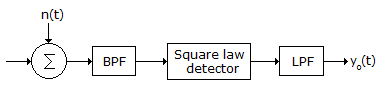XC(t) = AC[1 + u x (t)] cos ωct, |ux(t)| << 1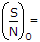if the channel noise is AWGN with PSD of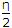A.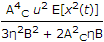B.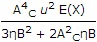C.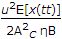D. None

Explanation:

No answer description available for this question. Let us discuss.

12.

In the given circuit, the switch is open for a long time. At time t = 0, the switch is closed. What are the initial and final values of voltages across the inductor?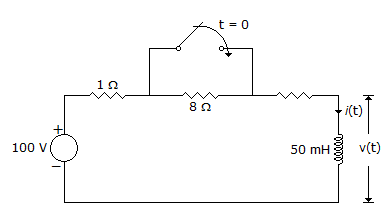A. 0 V and 0 V B. 0 V and 80 V C. 80 V and 0 V D. 80 V and 80 V

Explanation:

No answer description available for this question. Let us discuss.

13.

Consider the following statements:
In the case of space wave propagation, the signal strength at the receiver is

1. Directly proportional to transmitter and receiver heights.
2. Inversely proportional to distance between transmitter and receiver.
3. Directly proportional to frequency.
Which of the above statements is/are correct?

 A. 1 and 2 B. 1 and 3 C. 2 and 3 D. 3 only

Explanation:

No answer description available for this question. Let us discuss.

14.

An 8085 microprocessor is operating at 2 MHz frequency and executes the following program :
`START : MOV A, BOUT 55HDCR BSTA 2700HNOPNOPJMP START`
Identify the total number of machine cycles required to execute the above program till the JMP instruction is executed for the first time.

 A. 12 B. 13 C. 14 D. 15

Explanation:

No answer description available for this question. Let us discuss.

15.

An 8085 executes the following instructions`2710 LXI H, 30A0H2713 DAD H2714 PCHL`
All addresses and constants are in Hex. Let PC be the contents of the program counter and HL be the contents of the HL register pair just after executing PCHL.
Which of the following statements is correct?

 A. PC = 2715 H HL = 30A0 H B. PC = 30A0H HL = 2715H C. PC = 6104 H HL = 6140 H D. PC = 6140H HL = 2715H